# Four pavers

Four pavers would pave the square in 18 days. How many pavers do you need to add to done work in 12 days?

Result

n =  2

#### Solution:

4*18 = 12*(4+n)

12n = 24

n = 2

Calculated by our simple equation calculator.

Leave us a comment of example and its solution (i.e. if it is still somewhat unclear...):

Showing 3 comments:Idk
the answer should be 6,

the total "power" (as I like to call it) is 4 x 18, which is 72.
You divide that by 12, giving you six.

The calculator is wrong. Six pavers are needed.Dr Math
Six pavers are needed, but we need to add only 6-4=2 paversUser
Re @idk

4 * 18 = 12(4+n)
72 = 12(4+n)
-----------------
12
6 -4 =4 - 4+n
6 - 4 = n
2 = n#### To solve this example are needed these knowledge from mathematics:

Looking for help with calculating harmonic mean? Looking for a statistical calculator? Do you have a linear equation or system of equations and looking for its solution? Or do you have quadratic equation?

## Next similar examples:

1. Four workers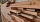Four workers will lay the floor of the gym in six hours. After an hour of working together, one of the workers left to the doctor. How long before the remaining three workers finish their work?
2. Reading recordsLucy, Tereza, and Petra decided to compile the compulsory reading records together. It would take 30 days for Lucia alone, 36 for Tereza and 45 for Petra. How long will take make records if they will work together?
3. Three peopleThree people start doing a work at a same time. The first worked only 2 hours. The second ended 3 hours before the end. On an individual basis, it would take the first time to do the work 10 hours, second 12 hours and 15 hours third. How many hours did it.
4. Find parametersFind parameters of the circle in the plane - coordinates of center and radius: ?
5. Loan 5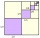Abdul takes a loan of 200000 from Ali and agrees to repay in number of instalment, each instalment begin with the 2nd exceeding the previous one by 1000, if the first instalment is 500, find how many instalment will be necessary to be wipe out the loan? C
6. Shopping center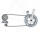The shopping center buys from the manufacturer bikes at a purchase price of 180 €. It sells them for a sale price of 250 €. However, in the advertising for the sale of these goods, the shopping center spent 20% of the selling price of all bicycles in stock
7. The King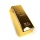The King wants to divide his sons equally. He has 42 rubies and 45 diamonds. How many sons and how will they share them?
8. The workThe work was to be done by 150 workers. At the beginning of their work, their number reduced by 40, which increased the time of work by 5 and 1/3 of the schedule. How long did work take?
9. Dog price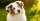Tereza agreed to get a dog and 6000 crowns a year for help in a dachshund breeding station. After eight months she had to finish work and got a dog and 2000 crowns. What price does a dog have?
10. Iron pole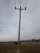The iron pole is in the ground 2/5 of its length, partly above the ground 1/3 is yellow, and the unpainted section is 6 m long. How long is the entire column?
11. Last pageTwo consecutive sheets dropped out of the book. The sum of the numbers on the sides of the dropped sheets is 154. What is the number of the last page of the dropped sheets?
12. Centroid - two bodiesA body is composed of a 0.8 m long bar and a sphere with a radius of 0.1m attached so that its center lies on the longitudinal axis of the bar. Both bodies are of the same uniform material. The sphere is twice as heavy as the bar. Find the center of gravit
13. Sand castle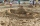Tim and Tom built a sand castle and embellished it with a flag. Half the pole with the flag plunged into the castle. The highest point of the pole was 80 cm above the ground, its lowest point 20 cm above the ground. How high was the sand castle?
14. Find the 9Find the missing angle in the triangle and then name triangle. Angles are: 95, 2x+15, x+3
15. MG=7x-15,MG=7x-15, FG=33, x=? Point M is the midpoint of FG. Find unknown x.
16. Midpoint 6FM=8a+1, FG=42, a=? Point M is the midpoint of FG. Find unknown a.
17. Midpoint 5FM=3x-4, MG=5x-26, FG=? Point M is the midpoint of FG. Use the given information to find the missing measure or value.